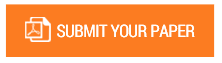A Numerical Statistical Solution to the Laplace and Poisson Partial Differential Equations

Authors : Dr. Ismail Mohamed Abbas

Volume/Issue : Volume 5 - 2020, Issue 11 - November

Scribd : https://bit.ly/2JIkcHs

Current work on the numerical solution of Laplace partial differential equation LPDE and of the Poisson partial differential equation PPDE is based on the replacement of the LPDE by an approximately equivalent system of n linear algebraic equations. The solution of this system has two distinct main approaches, namely direct methods and indirect or iterative techniques. In this article, we present a new statistical method based on the chain of recurrence relations of the so-called B matrix. Matrix B presents a chain recurrence relation where an algorithm for numerical calculations is simple. The correctness and precision of the numerical results are remarkable and superior to conventional methods. The proposed stochastic matrix B and its series of summations E are well defined and proven capable of handling the diversity of situations in different domains of LPDE and PPDE in 2D and 3D configurations such as the study of electrostatic voltage in the Poisson problem with Dirichlet boundary conditions. In this article, we explain the underlying theory and discuss in detail some 2D and 3D applications of the new numerical method

#### CALL FOR PAPERS

Paper Submission Last Date
31 - May - 2022